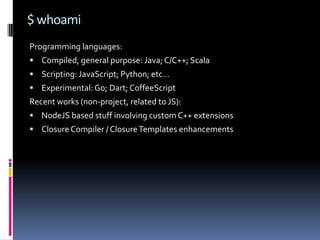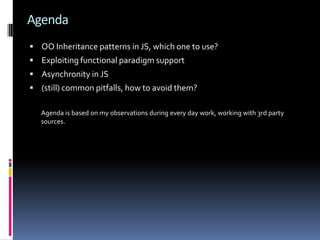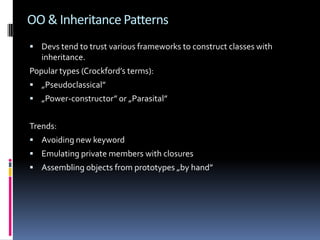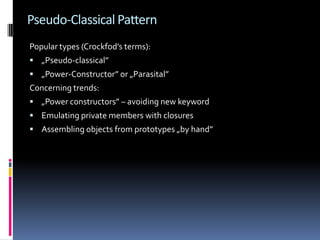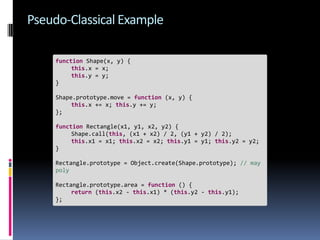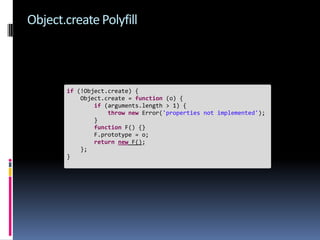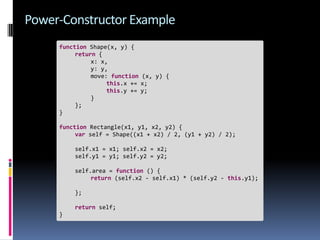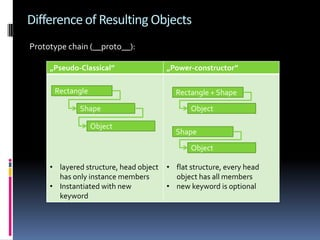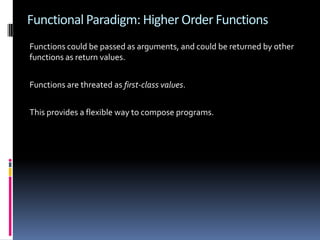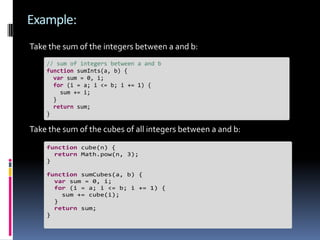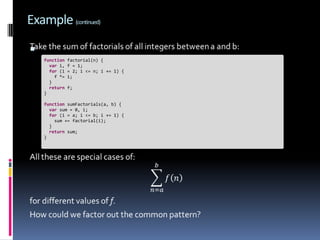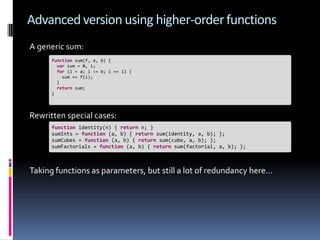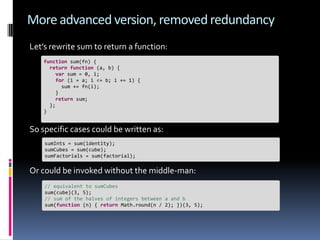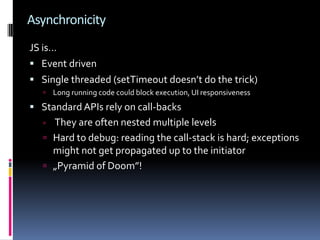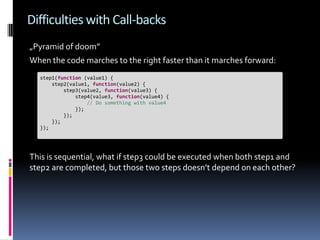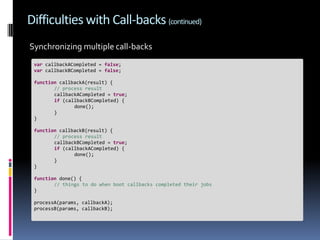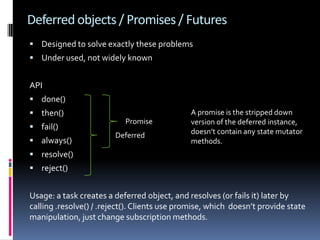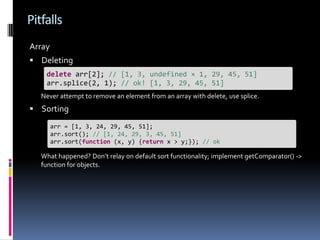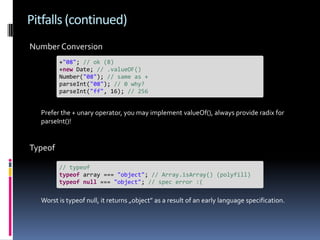1 of 23

2. \$ whoami Programming languages:  Compiled, general purpose: Java; C/C++; Scala  Scripting: JavaScript; Python; etc…  Experimental: Go; Dart; CoffeeScript Recent works (non-project, related to JS):  NodeJS based stuff involving custom C++ extensions  Closure Compiler / Closure Templates enhancements
3. Agenda  OO Inheritance patterns in JS, which one to use?  Exploiting functional paradigm support  Asynchronity in JS  (still) common pitfalls, how to avoid them? Agenda is based on my observations during every day work, working with 3rd party sources.
4. OO & Inheritance Patterns  Devs tend to trust various frameworks to construct classes with inheritance. Popular types (Crockford’s terms):  „Pseudoclassical”  „Power-constructor” or „Parasital” Trends:  Avoiding new keyword  Emulating private members with closures  Assembling objects from prototypes „by hand”
5. Pseudo-Classical Pattern Popular types (Crockfod’s terms):  „Pseudo-classical”  „Power-Constructor” or „Parasital” Concerning trends:  „Power constructors” – avoiding new keyword  Emulating private members with closures  Assembling objects from prototypes „by hand”
6. Pseudo-Classical Example function Shape(x, y) { this.x = x; this.y = y; } Shape.prototype.move = function (x, y) { this.x += x; this.y += y; }; function Rectangle(x1, y1, x2, y2) { Shape.call(this, (x1 + x2) / 2, (y1 + y2) / 2); this.x1 = x1; this.x2 = x2; this.y1 = y1; this.y2 = y2; } Rectangle.prototype = Object.create(Shape.prototype); // may poly Rectangle.prototype.area = function () { return (this.x2 - this.x1) * (this.y2 - this.y1); };
7. Object.create Polyfill if (!Object.create) { Object.create = function (o) { if (arguments.length > 1) { throw new Error('properties not implemented'); } function F() {} F.prototype = o; return new F(); }; }
8. Power-Constructor Example function Shape(x, y) { return { x: x, y: y, move: function (x, y) { this.x += x; this.y += y; } }; } function Rectangle(x1, y1, x2, y2) { var self = Shape((x1 + x2) / 2, (y1 + y2) / 2); self.x1 = x1; self.x2 = x2; self.y1 = y1; self.y2 = y2; self.area = function () { return (self.x2 - self.x1) * (self.y2 - this.y1); }; return self; }
9. Difference of Resulting Objects Prototype chain (__proto__): „Pseudo-Classical” „Power-constructor” Rectangle Rectangle + Shape Shape Object Object Shape Object • layered structure, head object • flat structure, every head has only instance members object has all members • Instantiated with new • new keyword is optional keyword
10. Which One is Better?  Power-Constructor pattern is extremely popular. A lot of frameworks using it, including jQuery.  Do we need privates? – not really  Performance? Demo time! Let’s evaluate performance with a particle system.
11. Conclusion Despite a little bit more verbose, might look not as self-contained than other variants, Pseudo-Classical inheritance is the way to go. Advantages:  Way batter performance, especially at large number of instances  Better IDE recognition  Better tooling (Closure-Compiler friendly)
12. Functional Paradigm: Higher Order Functions Functions could be passed as arguments, and could be returned by other functions as return values. Functions are threated as first-class values. This provides a flexible way to compose programs.
13. Example: Take the sum of the integers between a and b: // sum of integers between a and b function sumInts(a, b) { var sum = 0, i; for (i = a; i <= b; i += 1) { sum += i; } return sum; } Take the sum of the cubes of all integers between a and b: function cube(n) { return Math.pow(n, 3); } function sumCubes(a, b) { var sum = 0, i; for (i = a; i <= b; i += 1) { sum += cube(i); } return sum; }
14. Example (continued)  function factorial(n) { var i, f = 1; for (i = 2; i <= n; i += 1) { f *= i; } return f; } function sumFactorials(a, b) { var sum = 0, i; for (i = a; i <= b; i += 1) { sum += factorial(i); } return sum; }
15. Advanced version using higher-order functions A generic sum: function sum(f, a, b) { var sum = 0, i; for (i = a; i <= b; i += 1) { sum += f(i); } return sum; } Rewritten special cases: function identity(n) { return n; } sumInts = function (a, b) { return sum(identity, a, b); }; sumCubes = function (a, b) { return sum(cube, a, b); }; sumFactorials = function (a, b) { return sum(factorial, a, b); }; Taking functions as parameters, but still a lot of redundancy here…
16. More advanced version, removed redundancy Let’s rewrite sum to return a function: function sum(fn) { return function (a, b) { var sum = 0, i; for (i = a; i <= b; i += 1) { sum += fn(i); } return sum; }; } So specific cases could be written as: sumInts = sum(identity); sumCubes = sum(cube); sumFactorials = sum(factorial); Or could be invoked without the middle-man: // equivalent to sumCubes sum(cube)(3, 5); // sum of the halves of integers between a and b sum(function (n) { return Math.round(n / 2); })(3, 5);
17. Asynchronicity JS is…  Event driven  Single threaded (setTimeout doesn’t do the trick)  Long running code could block execution, UI responsiveness  Standard APIs rely on call-backs  They are often nested multiple levels  Hard to debug: reading the call-stack is hard; exceptions might not get propagated up to the initiator  „Pyramid of Doom”!
18. Difficulties with Call-backs „Pyramid of doom” When the code marches to the right faster than it marches forward: step1(function (value1) { step2(value1, function(value2) { step3(value2, function(value3) { step4(value3, function(value4) { // Do something with value4 }); }); }); }); This is sequential, what if step3 could be executed when both step1 and step2 are completed, but those two steps doesn’t depend on each other?
19. Difficulties with Call-backs (continued) Synchronizing multiple call-backs var callbackACompleted = false; var callbackBCompleted = false; function callbackA(result) { // process result callbackACompleted = true; if (callbackBCompleted) { done(); } } function callbackB(result) { // process result callbackBCompleted = true; if (callbackACompleted) { done(); } } function done() { // things to do when boot callbacks completed their jobs } processA(params, callbackA); processB(params, callbackB);
20. Deferred objects / Promises / Futures  Designed to solve exactly these problems  Under used, not widely known API  done()  then() A promise is the stripped down Promise version of the deferred instance,  fail() Deferred doesn’t contain any state mutator  always() methods.  resolve()  reject() Usage: a task creates a deferred object, and resolves (or fails it) later by calling .resolve() / .reject(). Clients use promise, which doesn’t provide state manipulation, just change subscription methods.
21. Demo
22. Pitfalls Array  Deleting delete arr; // [1, 3, undefined × 1, 29, 45, 51] arr.splice(2, 1); // ok! [1, 3, 29, 45, 51] Never attempt to remove an element from an array with delete, use splice.  Sorting arr = [1, 3, 24, 29, 45, 51]; arr.sort(); // [1, 24, 29, 3, 45, 51] arr.sort(function (x, y) {return x > y;}); // ok What happened? Don’t relay on default sort functionality; implement getComparator() -> function for objects.
23. Pitfalls (continued) Number Conversion +"08"; // ok (8) +new Date; // .valueOF() Number("08"); // same as + parseInt("08"); // 0 why? parseInt("ff", 16); // 256 Prefer the + unary operator, you may implement valueOf(), always provide radix for parseInt()! Typeof // typeof typeof array === "object"; // Array.isArray() (polyfill) typeof null === "object"; // spec error :( Worst is typeof null, it returns „object” as a result of an early language specification.